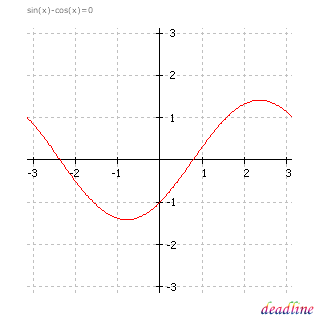# Solve trigonometric equations

Problem: solve a trigonometric equation

Equation to solve: sin(x)-cos(x) = 0

Interval for roots: [-3,14159265 ; 3,14159265]

DeadLine can solve trigonometric equations instantly, providing the equation graph and the roots.

Number of roots found: 2

x1 = -2,35619449

x2 = 0,78539816

The graph should seem familiar to you. Compare the roots of this trigonometric equation to the roots of: tan(x)=1.